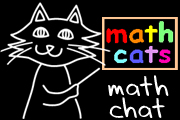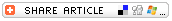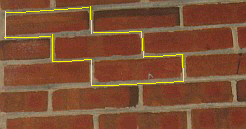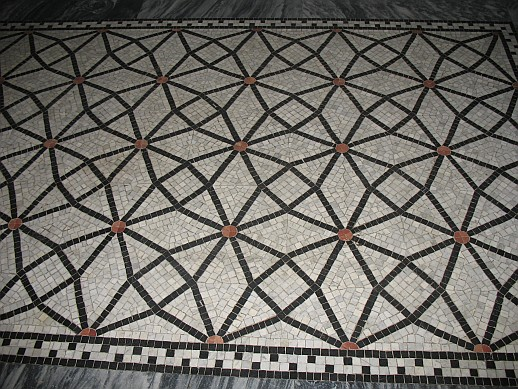## Search form# Functions in The Real WorldWhen we introduce students to functions, we typically bring the concept to life through the idea of function machines. But functions will really begin to come to life as our students find uses for functions in the real world.

## FUNCTION MACHINES

Students easily grasp the idea of a function machine: an input goes in; something happens to it inside the machine; an output comes out. Another input goes in; another output comes out. What's going on inside the machine? If we know the machine's function rule (or rules) and the input, we can predict the output. If we know the rule(s) and an output, we can determine the input. We also can imagine the machine asking, "What's my rule?" If we examine the inputs and outputs, we should be able to figure out the mystery function rule or rules.

e can make that metaphor even more concrete by setting up a large cardboard box with input and output slots. One student sits inside the function machine with a mystery function rule. As other students take turns putting numbers into the machine, the student inside the box sends output numbers through the output slot. After two or more inputs and outputs, the class usually can understand the mystery function rule.

 input output 4 12 5 15

"The function rule: Multiply by 3!"

Options for extending the activity include:

• Find the composite function (involving 2 or more function rules).
• Include fractions, decimals, and/or negative numbers.
• The teacher or the students can create spreadsheet function machines using the formula function.
• Students can create function tables for their classmates to solve, with one or two mystery function rules.

## ONLINE FUNCTION MACHINES

A number of wonderful online function machines develop the same concept. Students can work individually, in pairs, or as a class to solve the function machine puzzles.

• Math Playground's Function Machine
This machine for guessing mystery function rules lets the user control the maximum input number, with options for manual or computer inputs and 1 or 2 function rules. It requires five inputs/outputs before it will let the user guess the function rule(s).

• The three function machines at Shodor's Interactivate site include help and lessons for students and teachers:

• Annenberg Teachers' Lab Patterns in Mathematics: Mystery Operation
This challenging function machine takes user input for two variables to produce an output. After examining a series of input pairs and outputs, the user tries to deduce and apply the mystery operation to predict the output for a pair of machine-generated inputs. An example of a mystery operation in this machine is: a * (b 1).

## FUNCTIONS IN THE REAL WORLD

The NCTM Standard 2 for Patterns, Functions, and Algebra expects students to:

• understand various types of patterns and functional relationships;
• use symbolic forms to represent and analyze mathematical situations and structures;
• use mathematical models and analyze change in both real and abstract contexts.

The standards overview for grades 3-5 expects the understanding that "in the 'real-world,' functions are mathematical representations of many input-output situations."

As we point out and use functions in real-life settings, we can ask our students to keep alert for other input-output situations in the real world. You might draw from the following examples:

• A soda, snack, or stamp machine
The user puts in money, punches a specific button, and a specific item drops into the output slot. (The function rule is the product price. The input is the money combined with the selected button. The output is the product, sometimes delivered along with coins in change, if the user has entered more money than required by the function rule.)

• Measurement:

• Â ThermometerMost thermometers come with both Celsius and Fahrenheit scales. Students can study a thermometer as an input/output table. Students who have had experience in deducing composite function rules might be able to compare the two scales (noting, for instance, that 0Â° C = 32Â° F and 32Â° C = 50Â° F) and figure out the mystery function rule -- the formula for converting from one scale to the other.

• Circumference of a circle is a function of diameter.

Students can measure the diameter and circumference of several round containers or lids and record that data in a table. If diameter is the input and circumference is the output, what's the function rule?As they divide each container's circumference by its diameter to find that rule, they should notice a constant ratio -- a rough approximation of pi.

• Arm length is a function of height.
• Miles per gallon
A car's efficiency in terms of miles per gallon of gasoline is a function. If a car typically gets 20 mpg, and if you input 10 gallons of gasoline, it will be able to travel roughly 200 miles. The car's efficiency may be a function of the car's design (including weight, tires, and aerodynamics), speed, temperature inside and outside of the car, and other factors.

• Basic economics and money math:
• A weekly salary is a function of the hourly pay rate and the number of hours worked.
• Compound interest is a function of initial investment, interest rate, and time.
• Supply and demand: As price goes up, demand goes down.
• ShadowsThe length of a shadow is a function of its height and the time of day. Shadows can be used to find the height of large objects such as trees or buildings; the same function rule (ratio) by which we compare the length of an upright ruler to its shadow will help us find the unknown input (the height of the large object) when we measure its shadow.

• Geometric Patterns

• Polyhedra
As they examine the relationship between the number of faces, edges, and vertices of various polyhedra, can students discover Euler's rule? F + V = E + 2 (The number of faces plus the number of vertices equals the number of edges plus 2.) For example:
 Â # sides faces edges vertices per face tetrahedron 3 4 6 4 hexahedron 3 6 9 5 octahedron 3 8 12 6 decahedron 3 10 15 7 icosahedron 3 20 30 12 cube 4 6 12 8 dodecahedron 5 12 30 20

• Pattern block trains# of "cat heads" perimeter 1 7 2 12 3 17
Students can arrange a few pattern blocks in a simple design, give it a name, find the perimeter of the design (counting a standard edge as one unit), and then make a train of 2 or 3 repetitions of that design and find the perimeter of the train as it grows. As they make a data table, can they find a function rule or rules describing the relationship between the number of repetitions and the perimeter of the train?

The function rule: x5 +2 (or 5n + 2).

• Geometric patterns in the world around us
You or your students could take photos of architectural details with repeating patterns and make a table to analyze each pattern. For example, in the brick wall shown here, if each brick is 2" x 8", what is the perimeter of a series of bricks arranged in a stair-step pattern? What function rule describes that relationship?# of bricks perimeter 1 20 2 32 3 44

The function rule is x6 + 4 (or 6n + 4).

How about the patterns found in floor or wall tiles? What is the ratio of one color to another color? How many tiles of each color would we need to purchase to tile a room of a given size? What if we wanted to include a border of a given color? Some of the mosaic floor designs in the Library of Congress in Washington, D.C. are magnificent (and challenging) examples to study.Most of the examples in this article have featured data tables for analyzing functions, but of course, graphs are another effective means of representing input-output situations, including changes over time (whether the rate of change is constant or varying).

As students begin to use functions in the real world, more and more opportunities will present themselves, and the concept and value of functions will truly come to life.

Learning Math: Patterns, Functions, and Algebra
Annenberg Media has produced a fine collection of free online streaming videos on demand for teachers of grades K 8. Topics in this series include: algebraic thinking, patterns in context, functions and algorithms, proportional reasoning, linear functions and slope, solving equations, nonlinear functions, and classroom studies.

National Council of Teachers of Mathematics. (1998). Principles and standards for school mathematics. Reston, VA: NCTMWendy Petti is the creator of the award-winning Math Cats Web site, author of Exploring Math with MicroWorlds EX (LCSI, 2005), and a frequent presenter at regional and national math and technology conferences. She teaches grades 4 and 5 math at Washington International School.